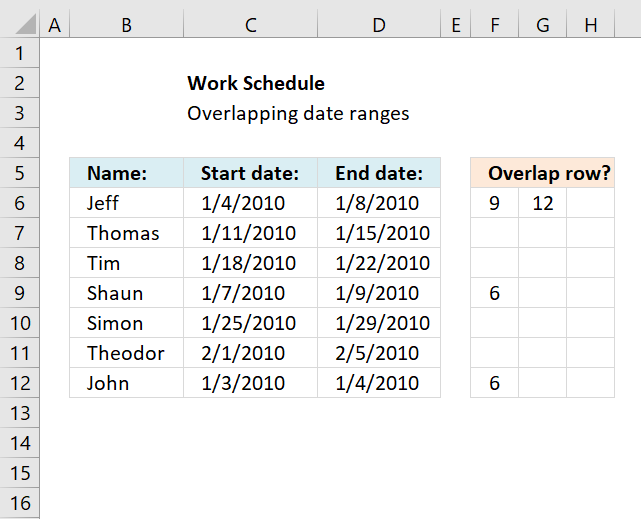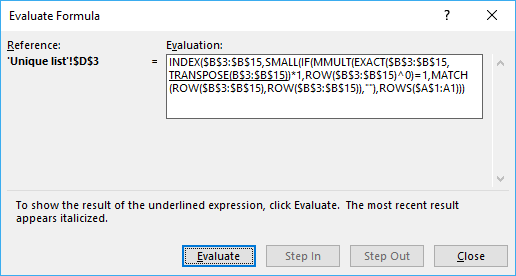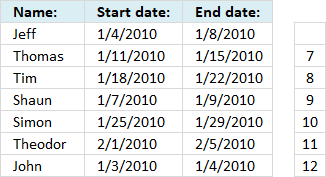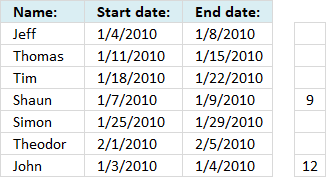Author: Oscar Cronquist Article last updated on July 11, 2018

Hi Oscar,

Great website! Keep up the good work.

I have a question as to how to expand this to the next step. Per your initial example we can see that Jeff in Row 1 and Shaun in Row 4 overlap their work schedule. I would like to know if there were a way, say for instance in Range E6:E12, to display Jeff’s Row number next to Shaun’s name and visa – versa. I can see how this will easily display what record numbers overlap when there is only one overlap in the range. Can we differentiate the records when there are two overlaps? Let’s say Theodor in Row 6 also has an overlap with Thomas in Row 2.

Now the data becomes confusing because we have to determine, from the 4 grayed rows, who overlaps with whom.

Can a formula return the Row value of the “matching” overlap record?
In Jeff’s E6 cell it would indicate “Row 4” as to the matching record, and the reverse for Shaun. Shaun’s E9 cell would indicate “Row 1” as the matching record.
And at the same time Theodor’s E11 cell would reflect “Row 2” for Thomas’s record and Thomas’ E7 cell would show “Row 6” for Theodor.

I haven’t even touched on the tougher one, such as what happens when John in Row 7 has an end date of 2010-01-07, thus overlapping with both Jeff and Shaun!

One step at a time. :)

ThanksThe array formula in cell F6 returns the rows of overlapping date ranges for the current record. The record on row 6 overlaps with both records on rows 9 and 12.

Records on row 9 and 12 show they overlap with the record on row 6, this makes it much easier to spot overlapping records.

Array formula in cell F6:

=IFERROR(SMALL(IF((\$C6<=\$D\$6:\$D\$12)*(\$D6>=\$C\$6:\$C\$12), IF(ROW(\$C\$6:\$C\$12)=ROW(), "", ROW(\$C\$6:\$C\$12)), ""), COLUMNS(\$A\$1:A1)), "")

How to enter an array formula

1. Copy above array formula (Ctrl + c)
2. Double click on cell F6
3. Paste above array formula (Ctrl + v)
4. Press and hold CTRL + SHIFT
5. Press Enter

How to copy array formula

1. Select cell F6
2. Copy cell F6 (Ctrl + c)
3. Select cell range F6:H6
4. Paste (Ctrl + v)
5. Select cell range F6:H6
6. Copy (Ctrl + c)
7. Select cell range F7:H12
8. Paste (Ctrl + v)

If you rather have row numbers concatenated in a single cell use the following formula.

=TEXTJOIN(", ", TRUE, IF((\$C6<=\$D\$6:\$D\$12)*(\$D6>=\$C\$6:\$C\$12), IF(ROW(\$C\$6:\$C\$12)=ROW(), "", ROW(\$C\$6:\$C\$12)), ""))

The TEXTJOIN function is available for Excel 365 subscribers, there is also UDF on the same webpage if you own an earlier version of Excel.

### Explaining array formula in cell F6

I recommend using the "Evaluate Formula" tool located on the Formula tab on the ribbon. It allows you to see each calculation step.#### Step 1 - Identify overlapping date ranges

The logical operators <> and = allows you to compare dates and find overlapping date ranges.

becomes

({TRUE; TRUE; TRUE; TRUE; TRUE; TRUE; TRUE})*({TRUE; FALSE; FALSE; TRUE; FALSE; FALSE; TRUE})

becomes

{1;0;0;1;0;0;1}

If you multiply boolean values you get this in Excel:

This is AND logic and the asterisk allows you to multiply arrays row-wise.

If you use the AND function it will apply AND logic to all values and return a single value, that is the reason we can't use it here.

We want it to return an array so we can easily identify the overlapping date ranges.

The array has the same number of values as there are date ranges, the position in the array corresponds to the position in the list of records.

#### Step 2 - Create an array containing row numbers except the current row number

To be able to return the correct row numbers we must create an array that is equally large as the previous array.

We don't want to return the date range for the current record so we need to figure out a way to remove the current row number from the array.IF(ROW(\$C\$6:\$C\$12)=ROW(), "", ROW(\$C\$6:\$C\$12)), "")

becomes

IF({6;7;8;9;10;11;12}=6, "", {6;7;8;9;10;11;12}, "")

returns

{"";7;8;9;10;11;12}

#### Step 3 - If current date range overlaps another date range then return row number

The logical expressions we built in step 1 is now used in an IF function to extract the correct row numbers of date ranges that overlap.IF((\$B6<=\$C\$6:\$C\$12)*(\$C6>=\$B\$6:\$B\$12), IF(ROW(\$B\$6:\$B\$12)=ROW(), "", ROW(\$B\$6:\$B\$12)), "")

becomes

IF({1;0;0;1;0;0;1}, {"";7;8;9;10;11;12}, "")

returns

{"";"";"";9;"";"";12}

#### Step 4 - Find the k-th smallest row number

The last step is to extract the smallest number based on where in worksheet the formula is calculated.

SMALL(IF((\$B6<=\$C\$6:\$C\$12)*(\$C6>=\$B\$6:\$B\$12), IF(ROW(\$B\$6:\$B\$12)=ROW(), "", ROW(\$B\$6:\$B\$12)), ""),COLUMN(A1))

becomes

SMALL({"";"";"";9;"";"";12}, COLUMN(A1))

The COLUMN function calculates the column number based on a cell reference. The cell reference A1 is relative meaning it changes when the cell is copied to cells to the right.

SMALL({"";"";"";9;"";"";12}, COLUMN(A1))

becomes

SMALL({"";"";"";9;"";"";12}, 1)

and returns 9 in cell F6.

In cell G6 the formula changes to

SMALL({"";"";"";9;"";"";12}, COLUMN(B1))

and becomes

SMALL({"";"";"";9;"";"";12}, 2)

and returns 12 in cell G6.